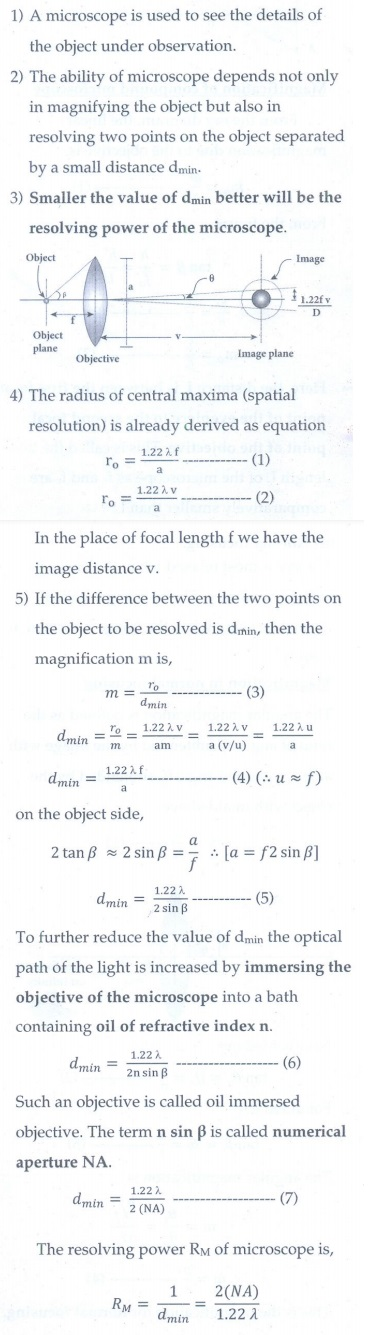Home | | Physics 12th Std | Long Answer Questions

## Chapter: 12th Physics : UNIT 7 : Wave Optics

Physics : Wave Optics: Book Back Questions, Answers, Solutions , Long Answer Questions

1. Prove laws of reflection using Huygens’ principle.2. Prove laws of refraction using Huygens’ principle.3. Obtain the equation for resultant intensity due to interference of light.4. Explain the Young’s double slit experimental setup and obtain the equation for path difference.5. Obtain the equation for bandwidth in Young’s double slit experiment.6. Obtain the interference in thin films and obtain the equations for constructive and destructive interference for transmitted and reflected light.7. Discuss diffraction at single slit and obtain the condition for nth minimum.8. Discuss the diffraction at a grating and obtain the condition for the mth maximum.9. Discuss the experiment to determine the wavelength of monochromatic light using diffraction grating.10. Discuss the experiment to determine the wavelength of different colours using diffraction grating.11. Obtain the equation for resolving power of optical instrument.12. Discuss about simple microscope and obtain the equations for magnification for near point focusing and normal focusing.13. Explain about compound microscope and obtain the equation for magnification.14. Obtain the equation for resolving power of microscope.16. Mention different parts of spectrometer and explain the preliminary adjustments.17. Explain the experimental determination of material of the prism using spectrometer.Tags : Wave Optics | Physics , 12th Physics : UNIT 7 : Wave Optics
Study Material, Lecturing Notes, Assignment, Reference, Wiki description explanation, brief detail
12th Physics : UNIT 7 : Wave Optics : Long Answer Questions | Wave Optics | Physics